Compatibility of temporal spectra with Kolmogorov (1941) and with random sweeping.

I previously wrote about temporal frequency spectra, in the context of the Taylor hypothesis and a uniform convection velocity of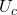, in my post of 25 February 2021. At the time, I said that I would return to the more difficult question of what happens when there is no uniform convection velcocity present. I also said that this would not necessarily be next week, so at least I was right about that.

As in the earlier post, we consider a turbulent velocity field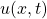which is stationary and homogeneous with rms value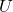. This time we just consider the dimensions of the temporal frequency spectrum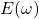. We use the angular frequency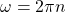, where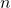is the frequency in Hertz, in order to be consistent with the usual definition of wavenumber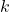. Integrating the spectrum, we have the condition:

(1)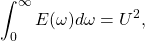which gives us the dimensions:

(2)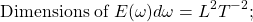or velocity squared.

For many years, the literature relating to the wavenumber-frequency correlation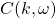has been dominated by the question: is decorrelation due to random sweeping effects, which would mean that the characteristic time is the sweeping timescale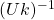; or is it characterised by the Kolmogorov timescale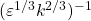? A recent article  makes a typical point about the consequences for the frequency spectrum of the dominance of the sweeping effect: ‘… the frequency energy spectrum of Eulerian velocities exhibits a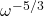decay, instead of the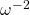expected from K41 scaling’. Which is counter-intuitive at first sight! As we saw in my blog of 26/02/21, for the case of uniform convectionis associated with K41.

Let us begin by clearing up the latter point. The authors of  cite the book by Monin and Yaglom, but I was unable to find it. (I mean the reference, not the book which is quite conspicuous on my bookshelves. I think that anyone giving a reference to a book, should cite the page number. Sometimes I do that and sometimes I forget!) In any case, it is easy enough to work out. From equation (2) we have the dimensions ofas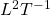. From the K41 approach we can write for the inertial range:

(3)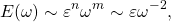where we fixed the dependence on the indexfirst.

The interest in random convective sweeping mainly stems from Kraichnan’s analysis of his direct-interaction approximation (DIA), dating back to 1959. A general discussion of this will be found in the book , but we can take a shortcut by noting that Kraichnan obtained an approximate solution for the reponse function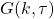of his theory (see page 219 of ) as:

(4)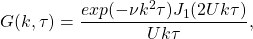where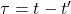,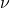is the kinematic viscosity, and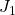is a Bessel function of the first kind. The interesting thing about this is that the K41 characteristic time for the inertial range does not appear. Also, in the inertial range, the exponential factor can be put to one, and the decay is determined by the sweeping time.

Corresponding to this solution for the inertial range, the energy spectrum takes the form:

(5)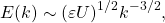as given by equation (6.50) in . As is well known, this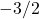law is sufficiently different from the observed form, which is generally compatible with the K41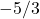wavenumber spectrum, to be regarded as incorrect. We can obtain the frequency spectrum corresponding to the random sweeping hypothesis by simply replacing the convective velocity, as used in Taylor’s hypothesis, by the rms velocity. From equation (8) of the earlier blog, we have;

(6)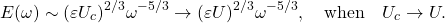This result is rather paradoxical to say the least. In order to get adependence on frequency, we have to have adependence on wavenumber! It is many years since I looked into this and in view of the continuing interest in the subject, I have begun to rexamine it. For the moment, I would make just one observation.

Invoking Taylor’s expression for the dissipation rate, which is: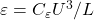, where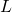is the integral lengthscale (not to be confused with the symbol for the length dimension) and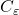asymptotes to a constant value for Taylor-Reynolds numbers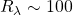, we may examine the relationship between the random sweeping and K41 timescales. Substituting for the rms velocity, have:

(7)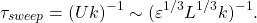Then, putting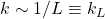, we obtain:

(8)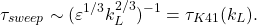So the random sweeping timescale becomes equal to the K41 timescale for wavenumbers in the energy-containing range. Just to make things more puzzling!

 A. Gorbunova, G. Balarac, L. Canet, G. Eyink, and V. Rossetto. Spatiotemporal correlations in three-dimensional homogeneous and isotropic turbulence. Phys. Fluids, 33:045114, 2021.
 W. D. McComb. The Physics of Fluid Turbulence. Oxford University Press, 1990.
 W. D. McComb, A. Berera, S. R. Yoffe, and M. F. Linkmann. Energy transfer and dissipation in forced isotropic turbulence. Phys. Rev. E, 91:043013, 2015.